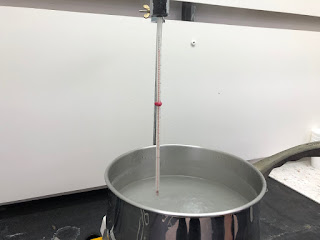## Wednesday, May 8, 2019

### Heat of Fusion and Heat of Vaporization

From kinetic theory, it is understood that as energy enters a substance, the molecules of the substance gain kinetic energy. Temperature is, in fact, defined as the average kinetic energy of the molecules of a substance.

As energy continues to flow into a substance, the kinetic energy of the molecules increase (that is to say the temperature goes up). This is true throughout the range of temperatures of the substance except at a few points: phase changes.

Heat of Fusion

When a substance reaches the melting point (heating up) or freezing point (cooling off), the temperature stops changing. When heating up, the added energy no longer increases the kinetic motion of the molecules but rather begins to break the bonds that hold a solid into a fixed shape and volume.

The amount of energy needed to break the bonds that holds a solid together is related to called the heat of fusion and is related to specific characteristics of the substance. Substances have different values for heat of fusion.

The amount of energy needed to break the bonds is also related to how much of the substance is present. Naturally, the more of something you have, the more energy it takes to melt it.

The total amount of heat needed to convert a substance from a solid to a liquid is determined by the value of the heat of fusion and how much of the substance is present, and can be found using the formula

Q = mΔHf

where Q is the heat (energy needed), m is the mass, and ΔHf is a number specific to each substance. ΔHis often provided in calories per gram, joules per gram, or joules per mole.

The process of going from liquid to solid work similarly, but in reverse. Instead of taking heat to break the bonds, the substance has to give off heat in order for the bonds to form. The amount is the same, and the same equation can be used.

Heat of Vaporization

In a similar way to that a substance goes from a solid to a liquid, the process of going from a liquid to a gas requires energy to break the bonds of the liquid that give a liquid its definite volume. The energy stops increasing the temperature of the substance, but instead go into breaking the bonds.This can easily be observed with a thermometer in a boiling liquid. No matter how long the pot sits on the heat source, the temperature of the water will only go so high—100° C—and after that, the energy from the heat source converts the water to a gas (steam).

How much heat is needed is, like melting, a function of the type of substance and the amount present. The more of a substance, the more energy needed to convert it all to a gas.

Thus, a formula emerges that is very like that for melting:

Q = mΔHv

where Q is the heat (energy needed), m is the mass, and ΔHv is a number specific to each substance. ΔHv is often provided in calories per gram, joules per gram, or joules per mole.

EXAMPLE

How much heat is needed to convert 8 grams of ice (solid) entirely to water (liquid) where ΔHf  for water is 333.55 J/g.

Q = mΔHv
Q = (8 gr )(333.55 J/g)
Q = (8 gr )(333.55 J/g)
Q = 2,688.4 J

Values for ΔHand  ΔHv can be found on many, many websites.